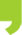## You are here

• You are here:
• Home > Events > Internal courses: Basics of Biostatistics 2022

# Internal courses: Basics of Biostatistics 2022

##Internal courses: Basics of Biostatistics 2022Application deadline: 1st of April 2022

Registration HERE

22/04/2022 01/07/2022

## Internal courses: Basics of Biostatistics 2022

On-line course

Description:

The course will give an overview of important concepts and methods used to analyse “Biomedical data”. The emphasis will be on the understanding of statistical concepts and their interpretation in a research framework. After a general introduction on probability theory and statistical inference, an emphasis will be made on common statistical methods. Particular cases will be used as illustrative examples.

The course will be composed of face-to-face theoretical lectures combined with practical sessions and additional short homework assignment.

Teacher: Hafid Laayouni (UPF)

Duration: 10 sessions (total 20h)

Schedule:  Each Friday from 22nd April (9:00-12:00h)  to 1st July 2022

Time: 10:00-12:00am

Where: Zoom

Requirements: Participants need to have basic R programing skills.

If you don't have it, you can do this course beforehand: https://biocorecrg.github.io/CRG_RIntroduction_2021/

Application deadline: 1st of April 2022

AGENDA:

• Introduction. Descriptive statistics. Probability and distributions. Sampling distribution. Confidence intervals - Theory 22nd April (9:00-12:00h)
• Hypothesis testing. The t-test - Theory - 29th April
• Exercises and Hands on sessions 1-2 - Practicum - 6th May
• Analysis of variance. One-way ANOVA - Theory - 13th May
• ANOVA. Multiple comparison procedures. Two-way ANOVA - Theory - 20th May
• Hands on sessions 3-4 - Practicum - 27th May
• Correlation and regression - Theory - 3th June
• Multiple regression analysis. Partial correlation - Theory - 10th June
• Hands on sessions 7-8 - Practicum - 17th June
• Effect Size and a summary of inference methods - Theory - 1st July

At the end of the course, the students will be able to:

• Express a scientific question in a mathematical formulation possible to analyse with statistical method.
• To be  confident about sampling methods and their impact on the quality of the data.
• Think on the basis of experimental design: replication, randomization and stratification.
• Think about the appropriate statistical method given the data we have (dependent and/or independent variable, continuous or categorical variables).
• Have a deep understanding of the meaning and interpretation of a test statistic and how to construct own statistic given data.
• Have a correct understanding of key words in basic statistic: null hypothesis, alternative hypothesis, error type I, error type II, statistical power, observational study, experimental study, causation and correlation, sampling error, random error, systematic error, sampling distribution, p-value, statistical and practical significance, effect size, statistical power.
• Correct interpretation of the result of a statistical test and been aware of the limitations of the applied methods.internal_training_bacis_of_biostatistics_2021_2.pdf